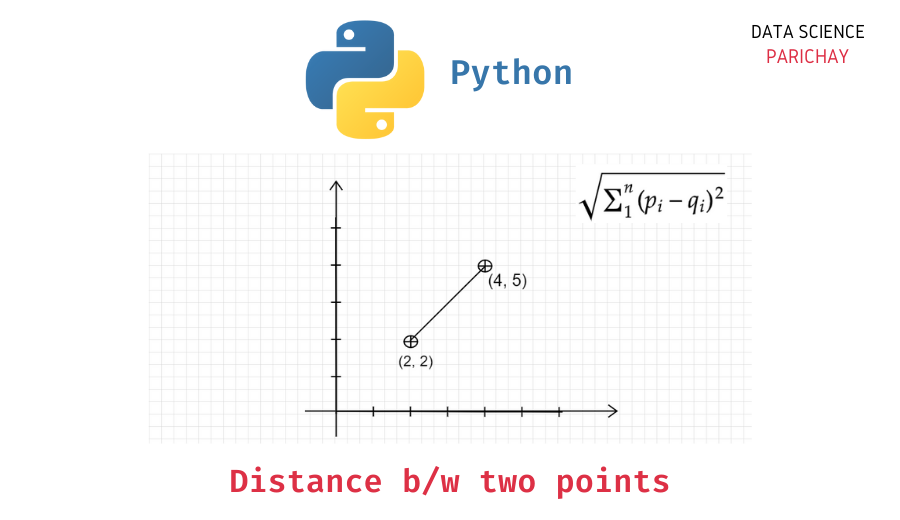# Calculate distance between two points in Python

In this tutorial, we will look at how to calculate the distance between two points in Python with the help of some examples.

If you prefer video over text, check out the following video detailing the steps in this tutorial –

There are a number of ways to compute the distance between two points in Python. You can compute the distance directly or use methods from libraries like `math`, `scipy`, `numpy`, etc.

We generally refer to the Euclidean distance when talking about the distance between two points. To calculate the Euclidean distance between the points (x1, y1) and (x2, y2) you can use the formula:

For example, the distance between points (2, 3) and (5, 7) is 5. Note that the above formula can be extended to n-dimensions.

Now that we know how the distance between two points is computed mathematically, we can proceed to compute it in Python.

Python has a number of libraries that help you compute distances between two points, each represented by a sequence of coordinates. Before we proceed to use off-the-shelf methods, let’s directly compute the distance between points (x1, y1) and (x2, y2).

```# point a
x1 = 2
y1 = 3
# point b
x2 = 5
y2 = 7

# distance b/w a and b
distance = ((x1 - x2)**2 + (y1 - y2)**2)**0.5
# display the result
print("Distance between points ({}, {}) and ({}, {}) is {}".format(x1,y1,x2,y2,distance))```

Output:

📚 Data Science Programs By Skill Level

Introductory

Intermediate ⭐⭐⭐

🔎 Find Data Science Programs 👨‍💻 111,889 already enrolled

Disclaimer: Data Science Parichay is reader supported. When you purchase a course through a link on this site, we may earn a small commission at no additional cost to you. Earned commissions help support this website and its team of writers.

`Distance between points (2, 3) and (5, 7) is 5.0`

You can see that we get the distance between the points (2, 3) and (5, 7) as 5. Note that the above formula works only for points in two dimensions.

Let’s now write a generalized function that can handle points with any number of dimensions.

```def get_distance(p, q):
"""
Return euclidean distance between points p and q
assuming both to have the same number of dimensions
"""
# sum of squared difference between coordinates
s_sq_difference = 0
for p_i,q_i in zip(p,q):
s_sq_difference += (p_i - q_i)**2

# take sq root of sum of squared difference
distance = s_sq_difference**0.5
return distance

# check the function
a = (2, 3, 6)
b = (5, 7, 1)
# distance b/w a and b
d = get_distance(a, b)
# display the result
print(d)```

Output:

`7.0710678118654755`

You can see that we used the function to get distance between two points with three dimensions each. We can now use this function to calculate distances between two points with any dimensions.

Note that the above function can further be improved by using vectorization to calculate the difference between the coordinates.

You can use the `math.dist()` function to get the Euclidean distance between two points in Python. For example, let’s use it the get the distance between two 3-dimensional points each represented by a tuple.

```import math

# two points
a = (2, 3, 6)
b = (5, 7, 1)

# distance b/w a and b
d = math.dist(a, b)
# display the result
print(d)```

Output:

`7.0710678118654755`

We get the same value as above.

The Euclidean distance is equivalent to the l2 norm of the difference between the two points which can be calculated in numpy using the `numpy.linalg.norm()` function.

```import numpy as np

# two points
a = np.array((2, 3, 6))
b = np.array((5, 7, 1))

# distance b/w a and b
d = np.linalg.norm(a-b)
# display the result
print(d)```

Output:

`7.0710678118654755`

We get the same result as above. Note that, here, we pass the difference between points a and b as a numpy array to the the `np.linalg.norm()` function.

The `scipy` library contains a number of useful functions of scientific computation in Python. Use the `distance.euclidean()` function available in `scipy.spatial` to calculate the Euclidean distance between two points in Python.

```from scipy.spatial import distance

# two points
a = (2, 3, 6)
b = (5, 7, 1)

# distance b/w a and b
d = distance.euclidean(a, b)
# display the result
print(d)```

Output:

`7.0710678118654755`

We get the same result as above. For more on the distance function, refer to its documentation.

With this, we come to the end of this tutorial. The code examples and results presented in this tutorial have been implemented in a Jupyter Notebook with a python (version 3.8.3) kernel having numpy version 1.18.5 and pandas version 1.0.5

•